• ## MIMO的信道容量以及实现

千次阅读 多人点赞 2019-09-28 14:39:25
所谓信道容量，是指信道能无差错的传输信息的最大信息速率，从信息论的角度，信道容量定义为： C=max⁡f(x) I(x,y)C=\underset{f(x)}{\mathop{\max }}\,I(x,y)C=f(x)max​I(x,y)      &...
最近听了老师讲MIMO信道容量的实现，感触良多，作为刚入门通信的菜鸟，特贴上老师上课的学习笔记，以此激励自己！
信道容量
所谓信道容量，是指信道能无差错的传输信息的最大信息速率，从信息论的角度，信道容量定义为：
$C=\underset{f(x)}{\mathop{\max }}\,I(x,y)$
其中$I(x,y)$是随机变量x和y的互信息，f(x)是x的概率密度函数，相当于，我们通过改变发射信号的概率密度函数，可以实现互信息的最大值，这个最大值就是信道容量。
SISO信道
先看最简单的单输入单输出信道模型：输出   $y(t)=hx(t)+z(t)$
通信原理我们学过，当输出x服从高斯分布时，可以达到信道容量：
$C={{\log }_{2}}(1+\frac{P}{{{\sigma }^{2}}}|h{{|}^{2}})$
这里对带宽进行了归一化，所以没有B。
MIMO信道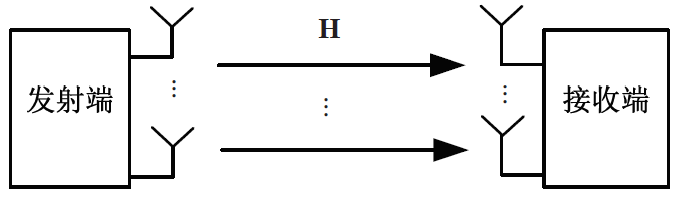输出   $y=Hx+z$
这里H是一个$r\times t$维的矩阵，输入x是一个$t\times 1$维的向量，t表示发射，r表示接收。z是一个服从均值为0，方差为${{\sigma }^{2}}I$的复高斯噪声。
回顾信息论，我们有如下公式：$I(x,y)=H(y)-H(y|x)$
这里x和y都是连续随机向量，H(y)是y的微分熵。
假设x和z相互独立，所以有H(y|x)=H(z)(个人理解是熵是不确定性的度量，x和z相互独立，所以x条件下y的熵，相当于求信道里面噪声的不确定性，也就是H(z)。
所以，求最大化互信息，当给定H(z)时，即为最大化H(y)，所以$I(x,y)=H(y)-H(z)$
当x服从复高斯分布，y服从复高斯分布时，y的微分熵最大。
$H(y)={{\log }_{2}}(\det (\pi e{{R}_{y}}))$$H(z)={{\log }_{2}}(\det (\pi e{{\sigma }^{2}}I))$
这里Ry是接收信号y的自相关矩阵。
${{R}_{y}}=E\{y{{y}^{H}}\}$ $=E\{(Hx+z){{(Hx+z)}^{H}}\}$ $=E\{(Hx{{x}^{H}}{{H}^{H}}+Hx{{z}^{H}}+z{{x}^{H}}{{H}^{H}}+z{{z}^{H}})\}$ $=HE\{x{{x}^{H}}\}{{H}^{H}}+{{\sigma }^{2}}I$ $=HS{{H}^{H}}+{{\sigma }^{2}}I$
这里，S是发送信号的协方差矩阵。因为x、z相互独立，所以乘积为0；信号的自协方差矩阵相当于方差，所以噪声那一项就变为了${{\sigma }^{2}}I$
代入熵的表达式中H(y)中，有：$I(x;y)={{\log }_{2}}\det (I+\frac{1}{{{\sigma }^{2}}}HS{{H}^{H}})$
所以，我们的目标就变为：$C=\underset{Tr(s)
如何设计发送信号的协方差矩阵S，使得上式最大？
采用方法：SVD分解，hadamard不等式，注水法
1、SVD分解
（要想知道公式怎么来的可以去参考矩阵论，这里不做推导）
对信道矩阵H进行SVD分解，有	$H=U\Lambda {{V}^{H}}$
其中，H的秩为m,U为$r\times m$,V为$t\times m$的矩阵，U、V都是酉矩阵，即装置乘以本身为单位阵。$\Lambda$为m*m维，其对角元素为$\lambda _{i}$非负。
所以高斯信道容量可以写为：
$C=\underset{Tr(s)定义$\hat{S}={{V}^{H}}SV$，再利用det(I+AB)=det(I+AB),可以得到：
$I(x;y)={{\log }_{2}}\det ({{I}_{r}}+\frac{1}{{{\sigma }^{2}}}U\Lambda {{V}^{H}}SV\Lambda {{U}^{H}})$$={{\log }_{2}}\det ({{I}_{m}}+\frac{1}{{{\sigma }^{2}}}{{V}^{H}}SV\Lambda {{U}^{H}}U\Lambda )$$={{\log }_{2}}\det ({{I}_{m}}+\frac{1}{{{\sigma }^{2}}}{{V}^{H}}SV{{\Lambda }^{2}})$$={{\log }_{2}}\det ({{I}_{m}}+\frac{1}{{{\sigma }^{2}}}\hat{S}{{\Lambda }^{2}})$
2、Hadamard不等式
Hadamard不等式：对任意半正定矩阵A的行列式小于或者等于所有对角元素的乘积：$det (A)\le {{\prod\limits_{i}{\left[ A \right]}}_{i,i}}$
当且仅当A是对角阵的时候成立。
所以，我们得到，$I(x;y)\le \sum\limits_{i=1}^{m}{{{\log }_{2}}\det (1+\frac{1}{{{\sigma }^{2}}}\lambda _{i}^{2}{{[\hat{S}]}_{i,i}})}$当且仅当${\hat{S}}$是对角阵的时候等号成立。
为了最大化互信息，假设${\hat{S}}={{V}^{H}}SV$=${{\Lambda }_{p}}$,即为对角矩阵。${{\Lambda }_{p}}$为功率分配矩阵${{\Lambda }_{p}}=diag\{{{p}_{1}},{{p}_{2}}...{{p}_{n}}\}$
我们有$S=V\hat{S}{{V}^{H}}$,代入I(X;Y)中，有：
$C=max I(x;y)=\sum\limits_{i=1}^{m}{{{\log }_{2}}(1+\frac{\lambda _{i}^{2}{{p}_{i}}}{{{\sigma }^{2}}})}$
**通过这个公式，对比siso的信道容量公式，就可以将mimo看成若干个siso的并行子信道，每个子信道的功率增益为$\lambda _{i}$。
同时，考虑发射功率会有约束，$Tr(S\le P)$，代入S，利用$Tr(AB=Tr(BA)$,我们有：
$Tr(S)=Tr(V{{\Lambda }_{p}}{{V}^{H}})=Tr({{\Lambda }_{p}}{{V}^{H}}V)=Tr({{\Lambda }_{p}})=\sum\limits_{i=1}^{m}{{{p}_{i}}}<=P$
我们这里通过S，反过来设计最优发射信号x ，使得上面的功率约束取等号，这里没细说，等我想明白了再写上
接下来，每个信号的强弱不一样，对每个信号分配不同的功率，会影响信道容量。该如何分配这个功率，可以使信道容量达到最大值呢？
3、注水法
注水算法的基本原理就是根据香农公式和限制条件，通过拉格朗日乘数法组成的一个方程，先令其偏导为零，求出Pi的表达式，但是Pi的表达式中包含一个未知数，再根据限制条件可以先求解出该未知数，再回代到之前的方程中，可以求解得每个信道根据信道质量分配得到的Pi。求解问题如下：
mimo信道容量：   max:    $C = \sum\limits_{i = 1}^m {{{\log }_2}\left( {1 + \frac{{{P_i}}}{{{\sigma ^2}}}{\lambda _i}} \right)}$
功率满足：              $\sum\limits_{i = 1}^m {{P_i} = P}$
上面为等式约束问题，可用拉格朗日乘子法求解。
引入拉格朗日函数，有  $Z(\lambda ,{P_i}) = \sum\limits_{i = 1}^m {{{\log }_2}\left( {1 + \frac{{{P_i}}}{{{\sigma ^2}}}{\lambda _i}} \right)} + L(P - \sum\limits_{i = 1}^m {{P_i}} )<=P$
对${{P_i}}$求偏导，有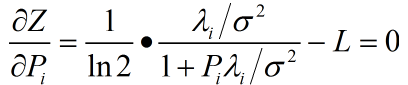解得：${P_i} = \frac{1}{{L \bullet \ln 2}} - \frac{{{\sigma ^2}}}{{{\lambda _i}}}=\mu-\frac{{{\sigma ^2}}}{{{\lambda _i}}}$
其中$\mu$为常数
因为功率不能为负数，将上述写为如下形式：
${P_i} = {\left( {\mu - \frac{{{\sigma ^2}}}{{{\lambda _i}}}} \right)^ + }$
所以最优的分配策略如上式
其中${\left( a \right)^ + } = \max (a,0)$
这里可用通过$\sum\limits_{i = 1}^m {{P_i}} = P = m\mu - \sum\limits_{i = 1}^m {\frac{{{\sigma ^2}}}{{{\lambda ^2}}}}$
得到：$\mu = \frac{{P + \sum\limits_{i = 1}^m {\frac{{{\sigma ^2}}}{{{\lambda ^2}}}} }}{m}$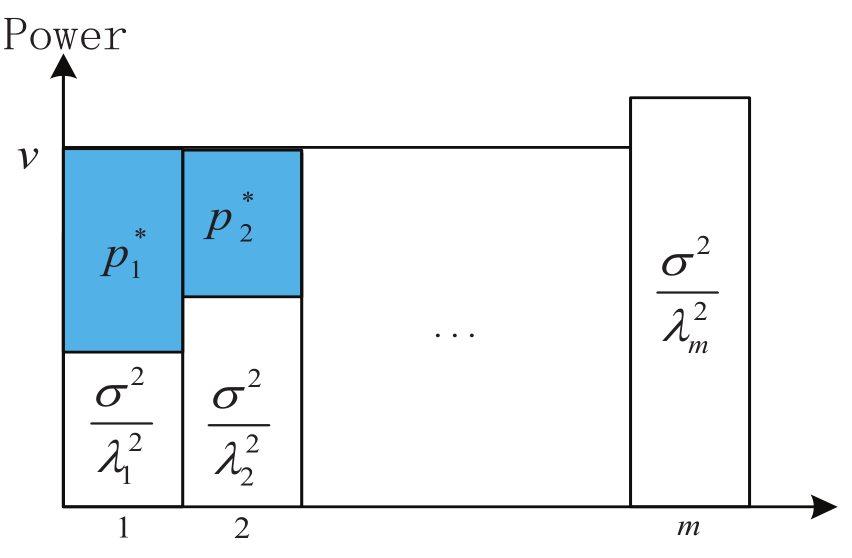也就是说，当已知csi（信道状态信息时），发送端会选择好的信道，给它分配大功率，过程如图示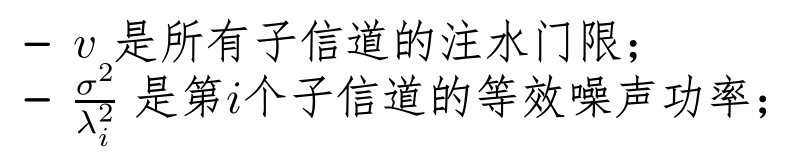时间有限，刚入门通信理论，不足之处我会虚心修改，打公式真的好难！


展开全文注水法
• 信道容量定义为信道输入与输出平均互信息的最大值。信道容量仅于信道特性，信道转移概率有关，与信道输入无关。但是信道输入概率必须与信道容量进行匹配才能达到容量。 2. 单符号离散信道 一个平稳离散无记忆信道...
1.    信道容量是信息能够可靠传输的最大传输速率。信道容量定义为信道输入与输出平均互信息的最大值。信道容量仅于信道特性，信道转移概率有关，与信道输入无关。但是信道输入概率必须与信道容量进行匹配才能达到容量。
2.    单符号离散信道
一个平稳离散无记忆信道的容量C定义为输入与输出平均互信息I(X;Y）的最大值，即
C=max I(X;Y）

C为信道传输最大信息速率能力的度量

3.    无损信道：每一个输入符号对应多个输出符号。C=logr。r为输入符号集的大小。

确定信道：每一个输出符号对应多个输入符号。C=logs。s为输出符号集的大小。

无损确定信道：每一个输入对应一个输出。 C= logs=logr;

4.    对称信道：信道转移概率矩阵按输出可以分为若干子集，每个子集都具以下特征：每一行是其他行的置换，每一列是其他列的置换，则信道成为准对称信道。

5.    转移概率矩阵可分为多个子集的对称信道称为准对称或弱对称信道。只有一个子集的信道称为强对称信道。

6.    对于离散对称信道，当输入等概率时达到信道容量，且信道容量为

C=H(Y)-H(p11,p12,……p1s).

7.    关于信道容量的注释：

1达到信道容量时的输入概率分布不唯一。

2达到容量时的输出概率严格为正。

3对应于信道容量的输出概率时唯一的。可以证明，如果两个输入概率达到同样容量，那么都对应着相同的输出分布。

展开全文• 在不同的《信息论》教材中，有关信道对称性的描述并不统一，这学习对称性信道的信道容量计算方法造成的一定障碍。因此，本文在文章的开篇部分将对本文中所描述的三种具有对称性的信道进行严格定义，以减小文章出现...
1. 三类具有对称性的信道
在不同的《信息论》教材中，有关信道对称性的描述并不统一，这为学习对称性信道的信道容量计算方法造成的一定障碍。因此，本文在文章的开篇部分将对本文中所描述的三种具有对称性的信道进行严格定义，以减小文章出现歧义的概率。

Symmetric Channel：
对称信道（Symmetric Channel）是最严格的对称性信道，该信道要求：

对于任意输入符号，其转移概率均为第一个输入符号转移概率的置换；即：
$\forall x_t\in X, \quad\exist \mathbf{E} = \prod \mathbf{E_{ij}},\quad s.t.\;\vec{p}(y|x_t) = \vec{p}(y|x_1)\mathbf{E}$
其中$\mathbf{E_{ij}}$是初等置换矩阵，$\vec{p}(y|x_t)$是第$t$个输入符号对应的转移概率向量，该向量是转移概率矩阵中的第$t$行。
对于任意输出符号，其转移概率均为第一个输出符号转移概率的置换；即：
$\forall y_t\in Y, \quad\exist \mathbf{E} = \prod \mathbf{E_{ij}},\quad s.t.\;\vec{p}(y_t|x) = \mathbf{E}\vec{p}(y_1|x)$
其中$\vec{p}(y_t|x)$是第$t$个输出符号对应的转移概率向量，该向量是转移概率矩阵中的第$t$列。

从信道矩阵观察，对称信道的任意一行的元素都完全相同，只是顺序发生了改变；任意一列也有相同的性质。

Wealky Symmetric Channel：
弱对称信道（Weakly Symmetric Channel）是对称信道的扩展，对称信道是弱对称信道的特例。相比对称信道，弱对称信道的条件约束更少，但性质却与对称信道基本相同。该信道要求：

对于任意输入符号，其转移概率均为第一个输入符号转移概率的置换；即：
$\forall x_t\in X, \quad\exist \mathbf{E} = \prod \mathbf{E_{ij}},\quad s.t.\;\vec{p}(y|x_t) = \vec{p}(y|x_1)\mathbf{E}$
其中$\mathbf{E_{ij}}$是初等置换矩阵，$\vec{p}(y|x_t)$是第$t$个输入符号对应的转移概率向量，该向量是转移概率矩阵中的第$t$行。
对于任意输出符号，其转移概率之和为常数；即：
$\forall y_t \in Y, \quad \sum_{x_i\in X}p(y_t|x_i) = C$

Quasi Symmetric Channel:
准对称信道（半对称信道，Quasi Symmetric Channel）是约束最弱的对称信道，对称信道与弱对称信道均是准对称信道的特例。该信道要求：

存在一种分块方式，$s.t.\;\mathbf{P} =[\mathbf{p_1},\mathbf{p_2},\cdots]$，且$\mathbf{p_1},\mathbf{p_2},\cdots$分块矩阵均满足对称信道条件。

下面列举的三个信道矩阵中，$\mathbf{P_1}$是对称信道，自然也属于弱对称信道和准对称信道；$\mathbf{P_2}$是弱对称信道，但也属于准对称信道；$\mathbf{P_3}$只是准对称信道。
$\mathbf{P_1} = \begin{bmatrix} 0.3 & 0.7\\ 0.7 & 0.3 \end{bmatrix}\quad \mathbf{P_2} = \begin{bmatrix} 0.4 & 0.1 & 0.2 & 0.3\\ 0.1 & 0.4 & 0.3 & 0.2 \end{bmatrix}\quad \mathbf{P_3}= \begin{bmatrix} 0.7 & 0.2 & 0.1\\ 0.2 & 0.7 & 0.1 \end{bmatrix}$
2. 对称性信道的信道容量计算
2.1. 对称信道
任何信道的平均互信息都可以写成信宿熵与噪声熵的差值，即：
$I(X;Y) = H(Y) - H(Y|X)$
噪声熵是一种条件熵，是条件事件$(y|x)$所对应的概率空间包含的信息量的期望，即：
$H(Y|X) = -\sum_{x_i\in X, y_j\in Y} p(x_i,y_j)\log_2p(y_j|x_i)$
考虑到期望运算的加权因子，即联合概率$p(x,y)$，实际上取决于信源概率分布和信道转移概率，即：
$p(x_i,y_j) = p(x_i) p(y_j|x_i)$
可以把噪声熵改写成另一种形式，在该形式中，信源分布和信道转移概率对噪声熵的影响被分离：
\begin{aligned} H(Y|X) &= -\sum_{x_i\in X, y_j\in Y} p(x_i,y_j)\log_2p(y_j|x_i)\\ &= -\sum_{x_i\in X}\sum_{y_j\in Y}p(x_i)p(y_j|x_i)\log_2p(y_j|x_i)\\ &= \sum_{x_i\in X}p(x_i)\left[ -\sum_{y_j\in Y} p(y_j|x_i)\log_2p(y_j|x_i) \right] \end{aligned}
考虑到对称信道的性质：对于任何输入符号，其转移概率分布都是第一个符号分布的置换，因此：
$\forall x_i\in X, \quad -\sum_{y_j\in Y} p(y_j|x_i)\log_2p(y_j|x_i) = C$
于是，信源分布不再对噪声熵有任何影响。噪声熵仅与信道转移概率有关，且转移概率矩阵的任意一行均可用来求解噪声熵：
\begin{aligned} H(Y|X) &= \sum_{x_i\in X}p(x_i)\left[ -\sum_{y_j\in Y} p(y_j|x_i)\log_2p(y_j|x_i) \right]\\ &= C\sum_{x_i\in X}p(x_i)\\ &= C\\ &= -\sum_{y_j\in Y} p(y_j|x_t)\log_2p(y_j|x_t) \quad \forall x_t \in X \\ \end{aligned}
于是，求解$I(X;Y) = H(Y) - H(Y|X)$在$\vec{p}(x)$上最大值的问题，转变为求解$H(Y)$在$\vec{p}(x)$最大值的问题。
信宿熵的本质也是一个概率空间的自信息熵。根据自信息熵的性质，当且仅当概率空间服从均匀分布时，自信息熵取得最大值。因此，可以试图让信宿分布达到均匀分布，进而使平均互信息最大。
需要注意的是，这样的尝试可能是失败的，因为信宿分布并不是一个可以调整的自变量。信宿分布是信源分布和信道转移概率作用后的结果，在给定信道的条件下，尝试让信宿服从均匀分布的过程就是在寻找一个合适的信源分布的过程。对于任意信道，这种信源分布很可能是不存在的（在后文求解准对称信道的章节中可以看到这一点）；但值得庆幸的是，对于对称信道，这种信源分布一定存在：
$\forall y_t\in Y,\quad p(y_t) = \sum_{x_i\in X}p(x_i,y_t) = \sum_{x_i\in X}p(x_i)p(y_t|x_i)$
需要注意的是，对称信道任意输出的转移概率分布都相同。即，只要$p(x_i)$与$x_i$无关，$\sum_{x_i}p(y_t|x_i)$就与$y_t$无关：
$p(y_t) = p(x_i)\sum_{x_i\in X}p(y_t|x_i)$
即：对于对称信道，当输入为均匀分布时，输出也是均匀分布。
综上，可以得出结论：对称信道的在输入为均匀分布时可以达到信道容量，信道容量的表达式为：
$C = \log_2r - H(Y|x_t)$
其中$r$是输出符号的数量，$H(Y|x_t)$是以任意一个输入符号为条件，输出符号空间的条件熵。
2.2. 弱对称信道
由于弱对称信道和对称信道均要求信道转移概率关于不同输入对称，因此，弱对称信道的噪声熵也与输入分布无关：
$H(Y|X)= -\sum_{y_j\in Y} p(y_j|x_t)\log_2p(y_j|x_t) \quad \forall x_t \in X$
因此，弱对称信道的信道容量也在信宿熵最大时取得。考虑到（2.1.）中求解信宿服从均匀分布时，只要求以下方程成立：
$p(y_t) = p(x_i)\sum_{x_i\in X}p(y_t|x_i)$
而弱对称信道的转移概率虽然关于不同输出并不对称，但其概率之和是定值，即$\sum_{x_i}p(y_t|x_i) = C$。因此，弱对称信道也能在均匀分布的信源作用下得到均匀分布的信宿。
因此，弱对称信道的信道容量与对称信道完全相同：弱对称信道在输入为均匀分布时可以达到信道容量，信道容量的表达式为：
$C = \log_2r - H(Y|x_t)$
其中$r$是输出符号的数量，$H(Y|x_t)$是以任意一个输入符号为条件，输出符号空间的条件熵。
2.3. 准对称信道
准对称信道的信道转移概率关于输入符号也是对称的，因此也具有对称信道和弱对称信道的关于噪声熵的结论：
$H(Y|X)= -\sum_{y_j\in Y} p(y_j|x_t)\log_2p(y_j|x_t) \quad \forall x_t \in X$
那么，求解信道平均互信息的最大值，理应也转化成求解信宿熵的最大值。与之前一样，我们期望存在一种信源分布，能使得信宿熵能实现均匀分布。然而令人遗憾的是，这种信源分布未必存在。
我们假设存在这样一种信源分布，能使信宿分布概率均为$1/r$，即：
$\begin{bmatrix} p(y_1|x_1) & p(y_2|x_1) & \cdots & p(y_r|x_1)\\ p(y_1|x_2) & p(y_2|x_2) & \cdots & p(y_r|x_2)\\ \vdots & \vdots & \ddots & \vdots\\ p(y_1|x_s) & p(y_2|x_s) & \cdots & p(y_r|x_s) \end{bmatrix}^T\times \begin{bmatrix} p(x_1)\\ p(x_2)\\ \vdots\\ p(x_s) \end{bmatrix}= \begin{bmatrix} 1/r\\ 1/r\\ \vdots\\ 1/r \end{bmatrix}$
能使输出达到均匀分布的信源分布是该线性非齐次方程组的一组解，但为了保证这个解确实能构成合理的信源概率空间，还需要添加概率空间完备性的约束条件$\sum_i p(x_i) = 1$，于是方程变为：
$\begin{bmatrix} p(y_1|x_1) & p(y_1|x_2) & \cdots & p(y_1|x_s)\\ p(y_2|x_1) & p(y_2|x_2) & \cdots & p(y_2|x_s)\\ \vdots & \vdots & \ddots & \vdots\\ p(y_r|x_1) & p(y_r|x_2) & \cdots & p(y_r|x_s)\\ 1& 1& \cdots & 1 \end{bmatrix}\times \begin{bmatrix} p(x_1)\\ p(x_2)\\ \vdots\\ p(x_s) \end{bmatrix}= \begin{bmatrix} 1/r\\ 1/r\\ \vdots\\ 1/r\\ 1 \end{bmatrix}$
该线性非其次方程组有解的充要条件是：系数矩阵与增广矩阵同秩。
未完。。。


展开全文• 考虑如下简单的加性高斯信道： 其中，X离散输入符号集X=[a0,a1,⋯ ,aM−1]X ...根据信道容量定义： C=max⁡{p(x=ai),i=1,2,...,M−1}{I(X;Y)}C = \mathop {\max }\limits_{\left\{ {p\left( {x = {a_i}} \right),
文章目录任意输入分布等概总结
任意输入分布
考虑如下简单的加性高斯信道：其中，X为离散输入符号集$X = \left[ {{a_0},{a_1}, \cdots ,{a_{M - 1}}} \right]$,由上图，$Y = X + n$。
根据信道容量的定义：
$C = \mathop {\max }\limits_{\left\{ {p\left( {x = {a_i}} \right),i = 1,2,...,M - 1} \right\}} \left\{ {I\left( {X;Y} \right)} \right\}$
可见，我们的目的是找到一个任意可能的输入信号的概率分布，使得最大化的互信息达到信道容量。如下图计算过程：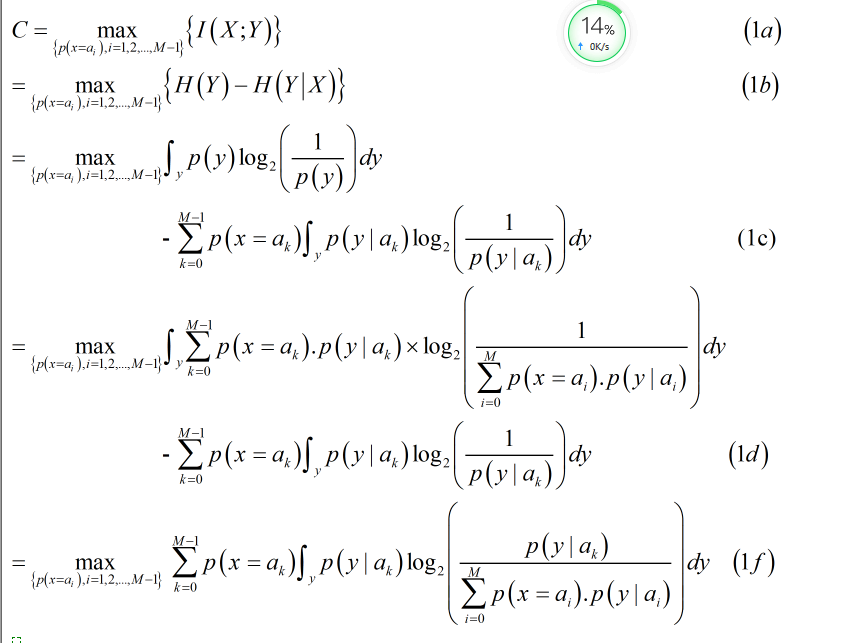其中，(1a)到(1c)是信息论课本中的相关定义，具体可百度；(1c)上半部分到(1d)是全概率公式的展开。
等概
假设输入符号是在M上是均匀分布的，
所以有：
$C = \frac{1}{M}\sum\limits_{k = 0}^{M - 1} {\int_y {p\left( {y|{a_k}} \right)} {{\log }_2}\left( {\frac{{p\left( {y|{a_k}} \right)}}{{\frac{1}{M}\sum\limits_{i = 0}^{M - 1} {p\left( {y|{a_i}} \right)} }}} \right)dy{\rm{ }}} （2）$
再用log(ab)=log(a)+log(b)与log(a/b) = -log(b/a)的性质，把上式分母中的1/M提出来，并将分数项倒过来，有：
$C = \frac{1}{M}{\log _2}M\sum\limits_{k = 0}^{M - 1} {\int_y {p\left( {y|{a_k}} \right)} } dy - \frac{1}{M}\sum\limits_{k = 0}^{M - 1} {\int_y {p\left( {y|{a_k}} \right)} } {\log _2}\left( {\frac{{\sum\limits_{i = 0}^{M - 1} {p\left( {y|{a_i}} \right)} }}{{p\left( {y|{a_k}} \right)}}} \right)dy{\rm{ }}（3）$
又因为,对该项$\int\limits_y {p\left( {y|{a_k}} \right)} dy$，其积分结果为1，这是因为归一性，即，y的所有概率和为1。所以有：
$C = {\log _2}M - \frac{1}{M}\sum\limits_{k = 0}^{M - 1} {\int_y {p\left( {y|{a_k}} \right)} } {\log _2}\left( {\frac{{\sum\limits_{i = 0}^{M - 1} {p\left( {y|{a_i}} \right)} }}{{p\left( {y|{a_k}} \right)}}} \right)dy{\rm{ }} （4）$
因为噪声是服从均值为0，方差为${\sigma ^2}$的高斯分布。对于p(y|ak)
有：
$p\left( {y|{a_k}} \right) = \exp \left( { - \frac{{{{\left| {y - {a_k}} \right|}^2}}}{{2{\sigma ^2}}}} \right) （5）$
将(5)带入(4)中，有：
$C = {\log _2}M - \frac{1}{M}\sum\limits_{k = 0}^{M - 1} {\int_y {p\left( {y|{a_k}} \right)} } {\log _2}\sum\limits_{i = 1}^{M - 1} {\exp \left( { - \frac{{{{\left| {y - {a_i}} \right|}^2} - {{\left| {y - {a_k}} \right|}^2}}}{{2{\sigma ^2}}}} \right)dy} （6）$
接着，令n=y-x,则dy=dz，y与z同号，故可进行积分代换,因为：故可以得到以下计算过程：其中，（6）到（7）是把y=x+n待入公式中，（7）到（8）是把积分写成数学期望的形式。（8）式的表达形式常可以在一些论文中见到。
总结

附：以上计算结果为单用户单天线的高斯信道，信道矩阵归一化为1了。可以参考经典论文，讨论的是单用户mimo高斯信道：

Globally Optimal Linear Precoders for Finite Alphabet Signals Over
Complex Vector Gaussian Channels

2.可以想象，如果是干扰信道的话，公式6中的y-ak不会再单纯的等于噪声n了，会引入干扰项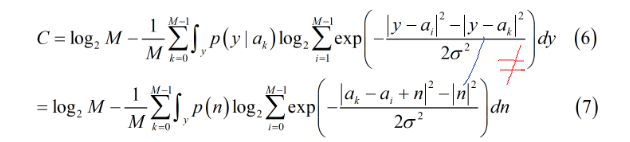具体是什么样的，后面我会再更新博客，也可以去搜一下关于广播和干扰信道的论文，其实就是广义二次矩阵相减的形式.
此外，在广播或者干扰信道的条件下，条件概率也不再单纯等于噪声，需要经过一点点小计算，最终的结果也会是用噪声来进行积分代换的。
敲公式不易，觉得有帮助的话麻烦点个赞把！


展开全文•       有效评估窄带阈下信道传递阈下信息的能力,对建立在密码学单向函数中的窄带阈下信道的...针对基于信息论的容量定义的不足,给出了基于误码率来计算信道容量的方法,具有更好的实用性。
• 当一个信道受到加性高斯噪声的干扰时，如果信道传输信号的功率和信道的带宽受限，则这种信道传输... 1、信道容量定义 在信息论中，称信道无差错传输信息的最大信息速率为信道容量，记。 从信息论的观点来看，
• matlab仿真mimo信道容量 SNR=15; %定义信噪比0dB disp('当nT=4时'); %显示接收天线数等于1 A=10^(SNR/10); %信噪比的单位转换关系式 Imin=eye(4); %"1"发射天线和接收天线两者数目较少的根...
• 信道容量定义为： C=I(X;Y)max⁡ C = I(X;Y)_{\max} C=I(X;Y)max​ 求解信道容量，即在以输入分布为自变量的情况下，求解平均互信息的极大值。需要注意的是：输入分布是信源概率空间中的概率，需要满足概率空间...数字通信 数学 矩阵
• 一些公式没法粘贴，以后再补上  一、用了matlab实现DMC容量迭代的算法如下： 设信道输入和输出符号集大小分别r,s. ...二、了解了信道容量定义和DMC信道容量迭代计算方法，我用了matlab来进行编程进行
• 根据极化码的原始论文中提出的信道容量为 它是一种信道速率的丈量,标志着信道速率的上限，，即信道的输入与输出的互信息量的最大值； 所谓的互信息定义是： 设两个随机变量的联合分布，边缘分布分别，互...
• 我们都是到对于任何一个信号经过傅里叶变换（Fourier Transition），可以在一个频率谱第一个零点定义为信号的带宽，常常被我们认为信号的基带带宽（Base bandwidth）。基带信号在发射过程中经过信号调制后根据信道的...
• 信道容量 一. 信息量 1. 定义 信息量是对事件的不确定性的度量，单位bit。 定义：在随机变量X中，事件x的(自)信息量I(X=x)I(X=x)I(X=x)简写I(x)=−log2p(x)I(x)=−log_2 p(x)I(x)=−log2​p(x)，单位bitbitbit。 ...数字通信
• 信道及其容量的问题 信道定义是广义的，可以指一段线路，也可以包含了设备的复杂系统。若信道的输入X=(X1,X2，......，Xi，......)，输出Y=(Y1,Y2，......，Yi，......)，通常定义条件概率p(Y|X)来描述信道...c
• 使用固定数量的信道为大量的、随机数量的用户提供服务。 在信道数量和呼叫阻塞概率之间进行折衷。 2、话务量与呼损 （1）话务量 度量话音通信中业务量的大小 ，其如同客流量。 呼叫话务量（流入话务量）：定义：...
• 限制码元在信道上的传输速率的因数有两个 1、 信道能够通过的频率范围 2、 信噪比 1.1奈氏准则 奈氏准则(无噪声情况下)：码元速率的极限值B与信道带宽W关系。...其定义为每秒钟传送码元的数目，...
• 目录： 缓冲信道声明 使用for循环读写信道 长度和容量 ... //声明缓冲信道a make初始化容量 a:=make(chan int,3) a<-1 a<-2 a<-3 //a<-4 fmt.Println(<-a) fmt.Println(...go
• 本文主要阐述了大规模... 我们使用预编码方法（包括零强制和最大比率合并方案）并优化功率分配以提高信道容量和传输速率。 此外，本文提供了数值结果以显示在单电池和多电池系统中的仿真性能，并扩展到了未来的前景。
• 本文提出了一个新概念-等效安全SNR被定义为清晰和简单。 并对正安全能力和安全性的存在概率进行了分析。 中断概率。 然后推导出人工噪声功率分配的最佳因子，并给出理论值。 窃听者的通道质量非常高时会出现这种...研究论文
• 现有的信道缩短均衡算法(如MMSE算法、MSSNR算法、MGSNR算法、MBR算法及Min-ISI算法等等)都定义了一个时延△，长度v+1的能量窗，其最优目标函数值都是在遍历最佳时延后得到的，通常最佳时延大于零。本文通过理论...
• 物理层安全容量定义为 Cs=max⁡PUX(I(U;Y)−I(U;Z)) C_s=\max_{P_{UX}}(I(U;Y)-I(U;Z)) Cs​=PUX​max​(I(U;Y)−I(U;Z)) 其中∣U∣≤∣X∣|U|\leq|X|∣U∣≤∣X∣. 当合法信道与窃听信道是degraded，或者more ...
• 结合过采样技术和香农限带高斯白噪声信道容量公式推导出了等效分辨率的公式，并以测量心电信号例，采样速率400 SPS、ADC参考电压2.5 V时，选用等效分辨率26位的ADC。经实例证明，等效分辨率ADC的性能...
• 摘要：为了给软件定义仪器中的数字化前端的设计和选择提供依据，提出了ADC等效分辨率的概念。结合过采样技术和香农限带高斯白噪声信道容量公式推导出了等效分辨率的公式，并以测量心电信号例，采样速率
• 结合过采样技术和香农限带高斯白噪声信道容量公式推导出了等效分辨率的公式，并以测量心电信号例，采样速率400SPS、ADC 参考电压2.5 V 时，选用等效分辨率26 位的ADC。经实例证明，等效分辨率ADC 的性能...
• 结合过采样技术和香农限带高斯白噪声信道容量公式推导出了等效分辨率的公式，并以测量心电信号例，采样速率400SPS、ADC 参考电压2.5 V 时，选用等效分辨率26 位的ADC。经实例证明，等效分辨率ADC 的性能...
• 在数字信道中，比特率是数字信号的传输速率，它用单位时间内传输的二进制代码的有效位(bit)数来表示，其单位每秒比特数bit/s(bps)、每秒千比特数(Kbps)或每秒兆比特数(Mbps)来表示(此处K和M分别1000和1000000，...
• 信道容量C定义为：互信息I(X；Y)的最大值 适用条件：离散无记忆信道 C = max I(X;Y) 简要介绍系统结构原理 通过NN1，先输出按照P(s)产生的0-15的symbol S，再将这些symbols变成星座点 x 通过NN2，接...深度学习 机器学习 tensorflow